# Logical Reasoning for Placement Test - Set 1

1)   In the following question, choose the correct code form.

If ‘air’ is called ‘green’, ‘green’ is called ‘red’, ‘red’ is called ‘sea’, ‘sea’ is called ‘blue’, ‘blue’ is called ‘water’ and ‘water’ is called ‘pink’, then what is the color of grass?

a. Green
b. Air
c. Red
d. Pink
 Answer  Explanation ANSWER: Red Explanation: The color of grass is green but green is called red.Hence, the color of grass is red.

2)   In the following question, choose the correct code form.

According to new terminology, ‘Aries’ means ‘air’, ‘Taurus’ means ‘light’, ‘Libra’ means ‘water’ and ‘Scorpio’ means ‘earth’. What would an organism breathe in?

a. Aries
b. Taurus
c. Libra
d. Scorpio
e. None of these
 Answer  Explanation ANSWER: Aries Explanation: One breathes in air.Air is called Aries as per the new terminology, so one would breathe in Aries.

3)   A team of 5 players Ashwin, Rahul, Sachin, Virat and Rohit participated in a tournament and played four matches.

The following table gives partial information about their individual scores and the total runs scored by the team in each match.

Each column has two values missing. These are the runs scored by the two lowest scorers in that match.

None of the two missing values is more than 10% of the total runs scored in that match.
Runs scoredMatch 1Match 2Match 3Match 4
Ashwin10053
Rahul886552
Sachin110
Virat72752056
Rohit6078
Total270300240200

What is the maximum possible percentage contribution of Ashwin in the total runs scored in the four matches?

a. 19.7%
b. 19.9%
c. 20.1%
d. 20.5%
 Answer  Explanation ANSWER: 19.7% Explanation: None of the two missing values is more than 10% of the total runs scored in that match.Maximum possible runs scored by Ashwin in Match-1 = 10% of 270 = 27Maximum possible runs scored by Ashwin in Match-3 = 19Note: Why is Ashwin’s score not 24? Because he has to score less than 3rd lowest scorer = 20So, Maximum possible percentage contribution:(27+100+19+53) / (270+300+240+200) x 100% = 199 / 1010 x 100% = 19.7%

4)   Choose the word which is least like the other words in the group.

b. Katana
c. Shotgun
d. Rifle
e. Revolver
 Answer  Explanation ANSWER: Katana Explanation: Here, all other weapons except Katana are firearms and can be used from a distance.

5)   Choose the word which is not similar to the other words in the group.

a. Peas
b. Cabbage
c. Spinach
d. Tomato
e. Potato
 Answer  Explanation ANSWER: Tomato Explanation: Here, all others except tomato are vegetables. Scientifically, tomato is a fruit. Hence, the answer is D.

6)   Solve the following question and choose the correct alternative from the following.
• 1, 2, 3, 4, and 5 are sitting in row but not necessarily in that order.
• All of them are sitting in a row with their backs toward North.
• 3 is immediate right to 5 and 4 is immediate left to 1. Only 2 is between 1 and 5.
Which of the following are at the extreme ends?

a. 3, 4
b. 2, 5
c. 2, 1
d. 4, 5

Explanation:
Since they have their back towards north i.e. they are facing south.

So, the left and right should be taken into consideration accordingly.

If a person is facing South, then, “Person’s Left” will be “Your Right” and vice-versa.

3 is immediate right to 5 i.e. 3, 5
4 is immediately left to 1 i.e. 1, 4

Since, 2 is between 1 and 5, so the sequence becomes 3, 5, 2, 1, 4

## 3 5 2 1 4

Thus, 3 and 4 are at extreme ends.

• A B C D E F are sitting in a row.
• E and F are in the center, A and B are at the ends.
• C is sitting on the left of A.
Who is sitting three places on the right of D?

a. B
b. C
c. A
d. D

Explanation:
C is on the left of A i.e. C, A
A and B are at the ends i.e. B, _, _, _, C, A
E and F are at the center i.e. B, _, E, F, C, A

So, the sequence becomes B D E F C A

## B D E F C A

C is three places the right of D.

8)   Complete the given series by finding the missing term

5, 15, 45, 225, ?

a. 1024
b. 1125
c. 1500
d. 2250
 Answer  Explanation ANSWER: 1125 Explanation: The pattern in the given series is (Number x 3) for first three positions then (Number X 5) for next positions. So, the next number should be: 225 x 5 = 1125

9)   Complete the given series by finding the missing term

28, 33, 31, ?, 34, 39

a. 34
b. 35
c. 36
d. 37
 Answer  Explanation ANSWER: 36 Explanation: The pattern in the given series is –+5 -2 +5 -2 +5 -2 ……The missing number should be – 31 + 5 = 36

10)   In the question below is given a statement followed by two courses of action numbered I and II. You have to assume everything in the statement to be true and based on the information given in the statement, decide which of the suggested courses of action logically follow(s) for pursuing.

(A) If only I follows
(B) If only II follows
(C) If either I or II follows
(D) If neither I nor II follow
(E) If both I and II follow.

Statement - Not just looks, internal beauty is extremely important.

Courses of Action - I. Pay attention to fashion.
II. Do not pay attention to fashion.
III. Books on self-development and spirituality should be encouraged.

a. Only I follows
b. Only II follows
c. Only III follows
d. Only I and III follow
e. Both I and II follow
 Answer  Explanation ANSWER: Only III follows Explanation: Course I and II lead to development or deterioration of outward appearance. The given statement speaks about the need for all-round personality development and a need to be a better individual.So, Only III follows.

11)   In the question below is given a statement followed by two courses of action numbered I and II. You have to assume everything in the statement to be true and based on the information given in the statement, decide which of the suggested courses of action logically follow(s) for pursuing.

(A) If only I follows
(B) If only II follows
(C) If either I or II follows
(D) If neither I nor II follow
(E) If both I and II follow.

Statement - Villages in Rajasthan have been severely damaged due to draught.

This has caused a financial burden on State Government.

Courses of Action - I. People of the area should be shifted to other suitable places.

II. State Government should ask for more financial support from Central Government.

a. Only I follows
b. Only II follows
c. Either I or II follows
d. Neither I nor II follow
e. None of these
 Answer  Explanation ANSWER: None of these Explanation: Since severe damage has been caused, people must be shifted to safer and suitable places for survival.Also, State Government should ask for more support from Central Government to meet up the expenses.Here, both the courses follow. But, there is no option as such, hence, the answer is E.

12)   In the question below is given a statement followed by two conclusions numbered I and II.

(A) If only I follows
(B) If only II follows
(C) If either I or II follows
(D) If neither I nor II follows
(E) If both I and II follow.

Statement - All men are mortal.

Conclusions - I. All must die one day.
II. Death can come at any place, any time.

a. Only I follows
b. Only II follows
c. Either I or II follows
d. Neither I nor II follows
e. Both I and II follow

13)   In the question below is given a statement followed by two conclusions numbered I and II.

(A) If only I follows
(B) If only II follows
(C) If either I or II follows
(D) If neither I nor II follows
(E) If both I and II follow.

Statement - Add colours to life - Use ‘CAMLIN’ colours.
Conclusions - I. Catchy slogans do not attract people.

II. Water colours are preferred by people.

a. Only I follows
b. Only II follows
c. Either I or II follows
d. Neither I nor II follows
e. Both I and II follow
 Answer  Explanation ANSWER: Neither I nor II follows Explanation: The slogan given in statement is a catchy one which implies catchy slogan do attract people. So, I does not follows.Nothing is given about preferences of people, so, II does not follow.

14)   Solve the following questions and choose the correct alternative from the following.
• Aman runs towards North for 10 m, turns left and runs another 20 m.
• He turns right and walks slowly for 10 m. Then he again turns right, walks another 50 m and stops.
In which direction is he with respect to his initial position?

a. South-East
b. North-East
c. North-West
d. South-West
 Answer  Explanation ANSWER: North-East Explanation: Tip: To answer the questions on this topic, always draw a rough diagram representing directions East, West, North, South, North-West, North-East, South-West, South-East. Now draw the diagram, as per the given instructions. As per the given directions, Aman runs 10 m north, turns left and runs another 20 m.Then, he turns right, walks another 10 m, again turns right and walks another 50 m.So, he is in North-East direction with respect to his initial position.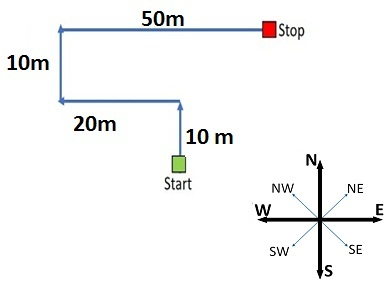15)   Solve the following question and choose the correct alternative from the following.
• Mohan is facing North and walks 20 km.
• He turns 270° anti-clockwise and walks 20 km.
• He again turns 45° clockwise and walks for 20 km.
Which direction is he facing now?

a. North-West
b. South-West
c. North-East
d. South-East
 Answer  Explanation ANSWER: South-East Explanation: Mohan walks 20 km north and turns 270° anti-clockwise which on calculation comes to right of his current position and walks another 20 km.He is currently facing East, then he turns 45° clockwise i.e. south-east direction and walks 20 km.Thus, he is facing south-east.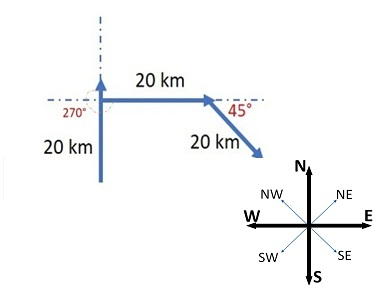16)   Pointing to an old woman, Aryan said ‘’Her son is my son’s uncle.”
How is Aryan related to old woman?

a. Mother
b. Sister
c. Son
d. Daughter
 Answer  Explanation ANSWER: Son Explanation: Aryan’s son → Son’s Uncle = Aryan’s Brother.So, the old woman’s son is Aryan’s brother i.e. the old woman is Aryan’s mother.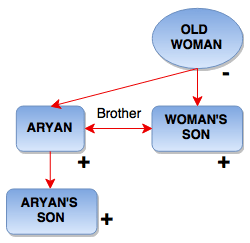17)   Pointing to a boy, a man said to a woman, “His mother is the only daughter of your father.”

How was the boy related to the woman?

a. Brother
b. Uncle
c. Husband
d. Son
 Answer  Explanation ANSWER: Son Explanation: Only daughter of woman’s father = Woman herselfMother of boy = Woman herselfSo, the woman is mother of the boy.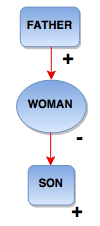18)   Pointing to a photograph the man said, “The lady in the photograph is my nephew’s maternal grandmother.”

How is the lady in the photograph related to the man’s sister who has no other sister?

a. Cousin
b. Sister-in-law
c. Mother
d. Mother-in-law
 Answer  Explanation ANSWER: Mother Explanation: Man’s sister → Sister’s son = NephewNephew’s mother = Man’s sisterMother of man’s sister = Maternal GrandmotherSo, the lady in the photograph is mother of man’s sister.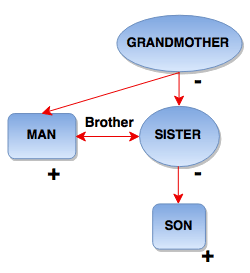19)   How many times do the hands of a clock coincide in 24 hours?

a. 22
b. 24
c. 26
d. 28
 Answer  Explanation ANSWER: 22 Explanation: The hands of a clock coincide 11 times in every 12 hours (Since between 11 and 1, they coincide only once, i.e. at 12 o'clock).The hands coincide 22 times in a day.

20)   How many times are the hands of a clock at right angles in 24 hours?

a. 22
b. 44
c. 24
d. 48
 Answer  Explanation ANSWER: 44 Explanation: In 12 hours, they are at right angles 22 times.In 24 hours, they are at right angles 44 times.